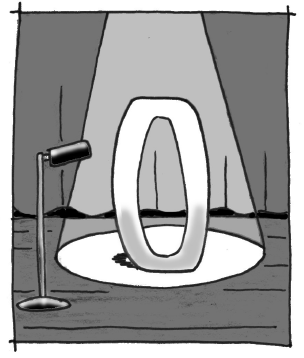### Home > AC > Chapter 8 > Lesson 8.2.2 > Problem8-55

8-55.

1. Find each sum:

$0+3 =$

$-7+0=$

$0+6=$

$0+\left(-2\right)=$

1.What is special about adding zero? Write a sentence that begins, “When you add zero to a number, …”

1. Julia is thinking of two numbers $a$ and $b$. When she adds them together, she gets a sum of $b$. Does that tell you anything about either of Julia’s numbers?

What did you notice about each of the answers in part (a)?

2. Find each product:

$3\cdot0=$

$\left(-7\right)\cdot0=$

$0\cdot6=$

$0\cdot\left(-2\right)=$

1. What is special about multiplying by zero? Write a sentence that begins, “When you multiply a number by zero, …”

When you multiply a number by zero, … the result is always $\mathit{0}$.